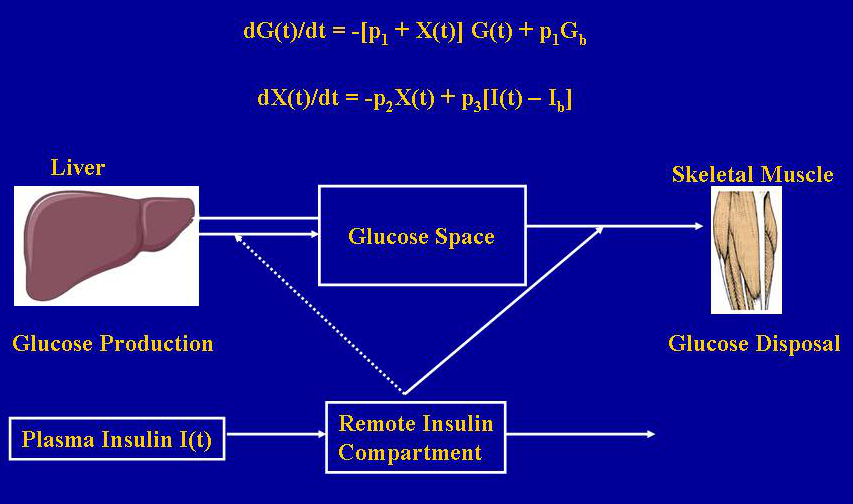# endo ch5f3Figure 3. Schematic, equations, and parameters for the minimal model of glucose metabolism. Differential equations describing glucose dynamics (G(t)) in a monocompartmental “glucose space” and insulin dynamics in a “remote compartment” (X(t)) are shown at the top. Glucose leaves or enters its space at a rate proportional to the difference between plasma glucose level, G(t) and the basal fasting level, Gb. In addition, glucose also disappears from its compartment at a rate proportional to insulin levels in the “remote” compartment (X(t)). In this model, t = time; G(t) = plasma glucose at time t; I(t) = plasma insulin concentration at time t; X(t) = insulin concentration in “remote” compartment at time t; Gb = basal plasma concentration; Ib = basal plasma insulin concentration; G(0) = G0 (assuming instantaneous mixing of the IV glucose load); p1, p2, p3, and G0 = unknown parameters in the model that are uniquely identifiable from FSIVGTT; glucose effectiveness, SG = p1; and insulin sensitivity, SI = p3/p2..